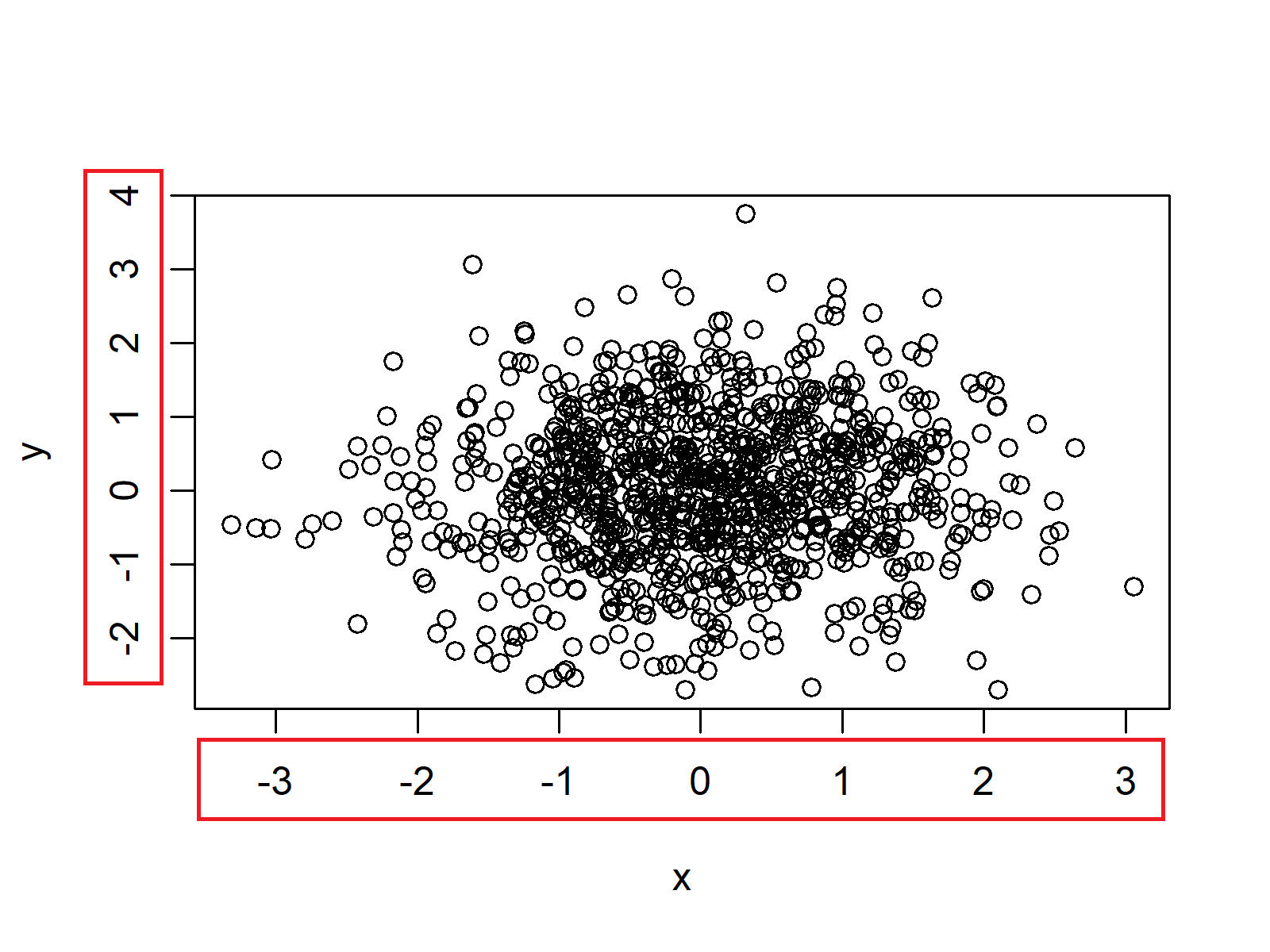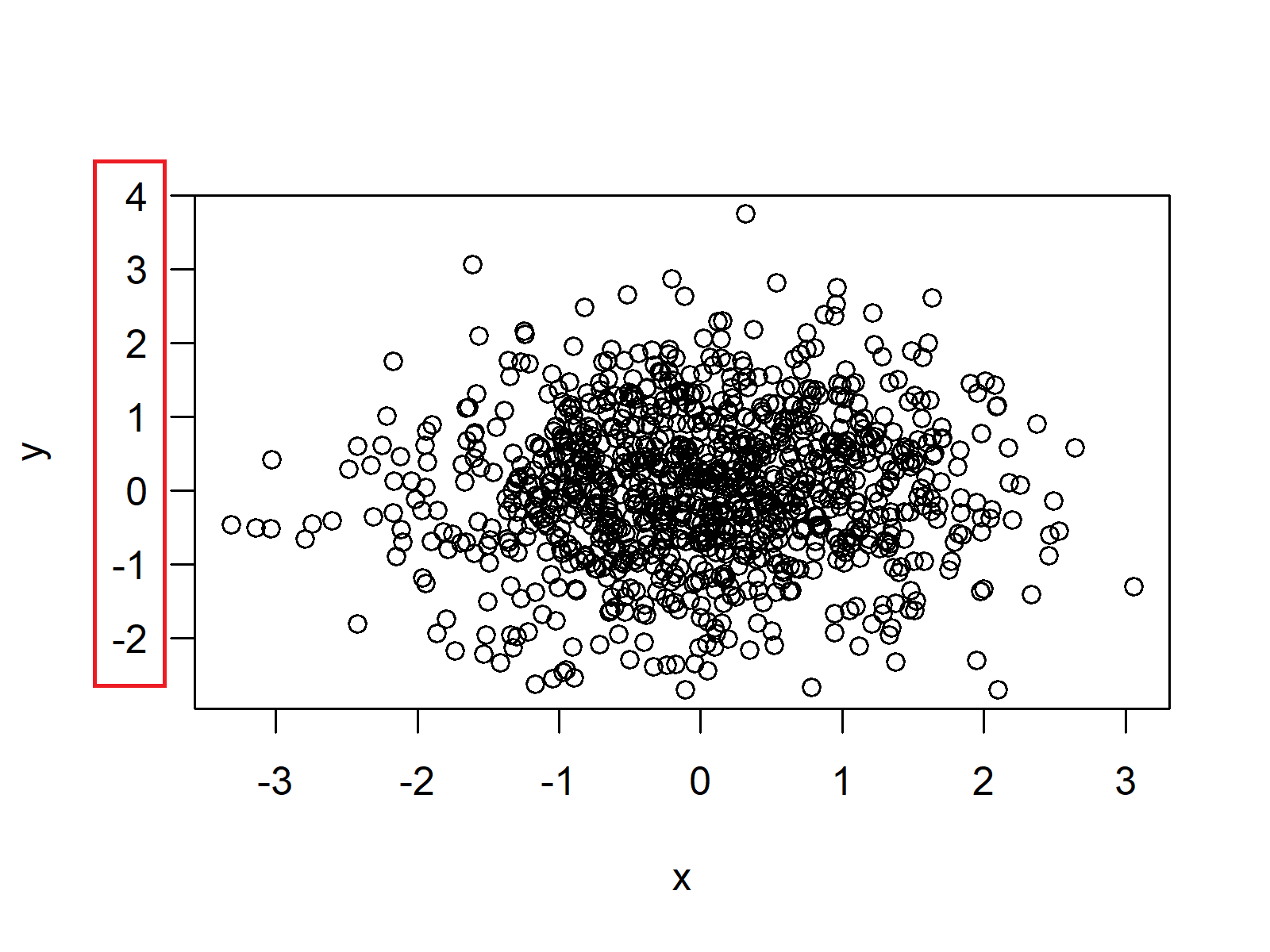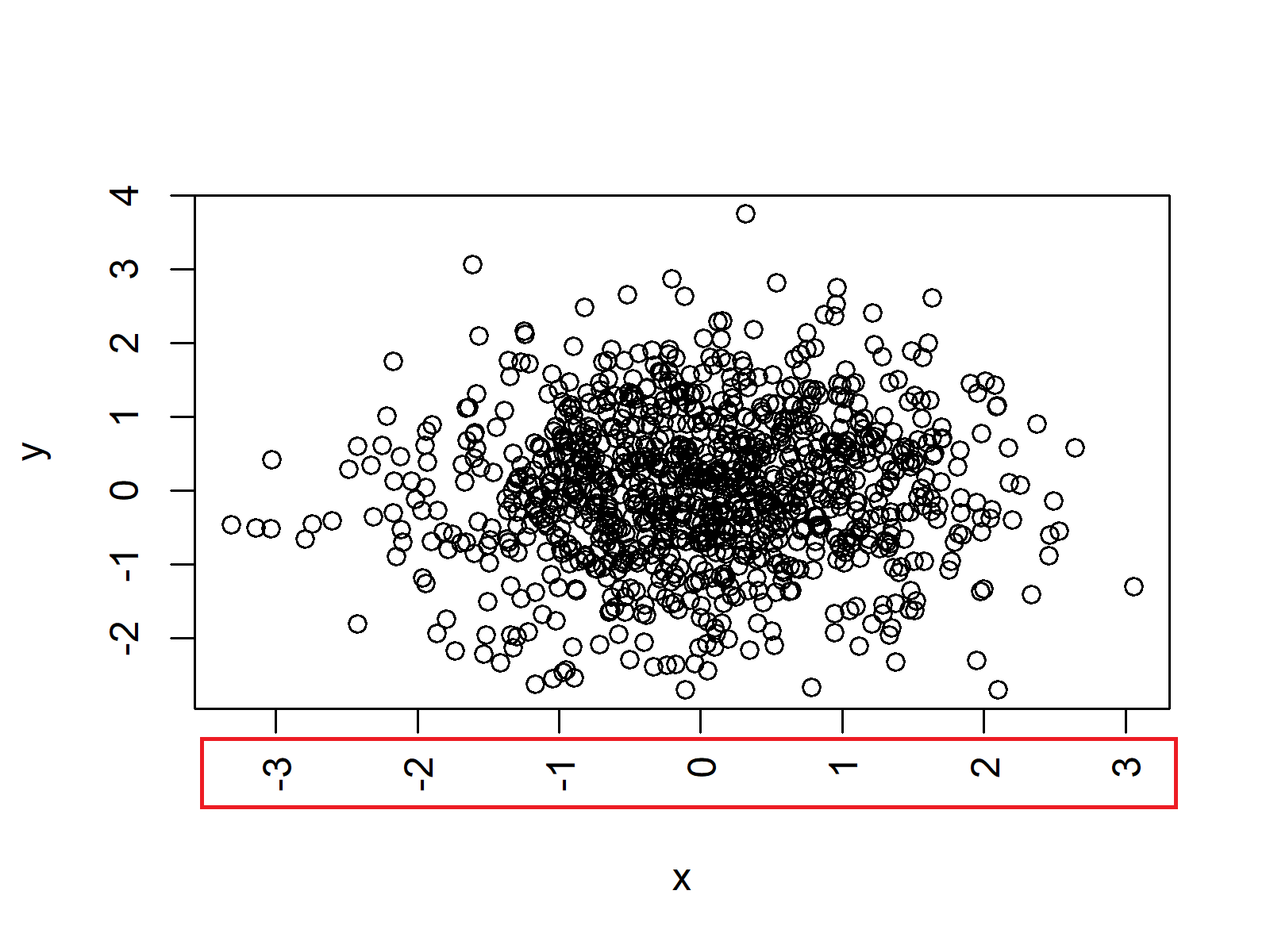# Rotate Axis Labels of Base R Plot (3 Examples)

In this article, I’ll explain how to rotate axis labels of a Base R plot in the R programming language.

The content of the post is structured as follows:

Let’s get started…

## Example Data

In the examples of this R tutorial, we’ll use the following example data:

```set.seed(77777)        # Create example data
x <- rnorm(1000)
y <- rnorm(1000)```

The default plot looks as follows:

`plot(x, y)             # Default plot - parallel to the axis`Figure 1: Base R Plot with Default Specifications.

The axis labels of the x-axis have a horizontal orientation and the y-axis labels have a vertical orientation.

## Example 1: Rotate Axis Labels Horizontally

In order to change the angle of the axis labels of a Base R plot, we can use the las argument of the plot function. If we want to rotate our axis labels to a horizontal position, we have to specify las = 1:

`plot(x, y, las = 1)    # Horizontal labels`Figure 2: Horizontal Angle of Axis Labels.

Note that we can modify the las argument in any kind plot of Base R. In this example, we illustrated the las argument based on a scatterplot. However, we could also apply this R syntax for a barplot, histogram, boxplot, and so on…

## Example 2: Rotate Axis Labels Perpendicular to the Axis

If we want to show our axis labels in a perpendicular angle to the Axis, we have to specify las = 2:

`plot(x, y, las = 2)    # Perpendicular to the axis`Figure 3: Labels Perpendicular to the Axis.

## Example 3: Rotate Axis Labels Vertically

For vertical axis labels, we need to specify las = 3:

`plot(x, y, las = 3)    # Vertical labels`Figure 4: Vertical Angle of Axis Labels.

## Video, Further Resources & Summary

Have a look at the following video of my YouTube channel. In the video, I explain the R programming syntax of this article in R:

In addition to the video, you could read the related articles on https://statisticsglobe.com/.

In this post you learned how to adjust the angles of axis text with a different degree of rotation in R programming. Don’t hesitate to let me know in the comments, if you have any further questions.

Subscribe to the Statistics Globe Newsletter